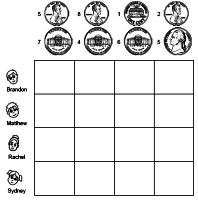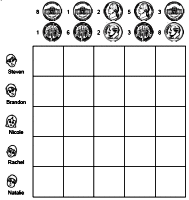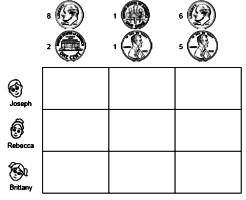edHelper.com Life Skills Writing Checks Math Worksheets Money Worksheets, Printables, Activities, and Lesson Plans Money Word ProblemsWorksheets, Lessons, and Printables

3-Digit Addition and Subtraction
Problem solving: Money word problems

Problem solving

Logic Puzzles: Coins - EasierPennies and nickels Nickels and dimes Pennies, Nickels, or Dimes (each key will use pick two coins)

Logic Puzzles: Coins - Greater and Less than CluesPennies and nickels Nickels and dimes Pennies, Nickels, or Dimes (each key will use pick two coins)

Logic Puzzles: Coins - Comparing Total Value of CoinsPennies and nickels Nickels and dimes Pennies, Nickels, or Dimes (each key will use pick two coins)

Word Problems
Addition and Subtraction Word Problems (Grades Three-Five)
Addition and Multiplication Word Problems (Grades Five-Six)
Multiplication and Division Word Problems (Grades Five-Seven)
All of the above Word Problems in a CrossNumber Puzzle (Grades Five-Seven)

Interest Worksheets
Interest Word Problems (Difficult)

Algebra Money Word Problems

Money

Have a suggestion or would like to leave feedback?